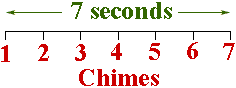Quandaries and Queries Dear Q and Q,   I have a math problem that I want you to solve. here it goes:   A clock chimes 7 times in 7seconds.  how long does it take the clock to chime 10 times? Hi, I would draw a time line, 7 seconds long, and mark the chimes, equally spaced along the line.The 7 chimes divide the 7 seconds into 6 time intervals of equal length. How long is each of these time intervals? Now chimes 8, 9 and 10. Penny Go to Math Central